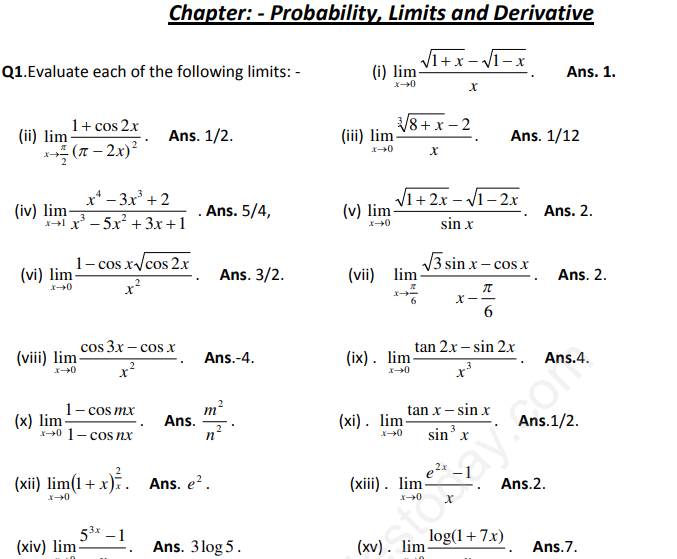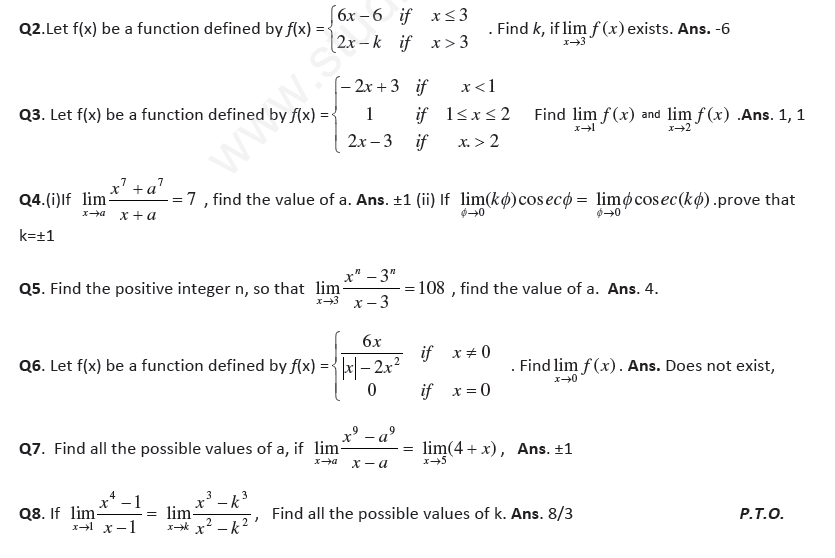CBSE Class 11 Probability

• NCERT Solutions Class 11 Maths Probability

NCERT Solutions Class 11 Maths Probability. The NCERT solutions for Class 11 Mathematics have been made by Mathematics teacher of one of the best CBSE school in India. These NCERT solutions have been made to give detailed answers and expanations of the concepts as per NCERT which can be easily...

• CBSE Class 11 Mathematics Probability Assignment Set A

Probability MCQ Questions with Answers Class 11 Mathematics   Q.- A binary number is made up of 8 bits. The probability of an incorrect bit appearing is p and the errors in different bits are independent of one another. The probability of forming an incorrect number is (a) P/8 (b) p8 (c) 1 – (1 –...

• CBSE Class 11 Mathematics Probability Assignment Set B

Q14. The letter of the word ‘SOCIETY’ are placed at random in a row. What is the probability that the three vowels come together. Ans. 1/7 Q15.Four digit numbers are formed by using the digit 1,2,3,4 and 5 without repeating any digit. Find the probability that a number, chosen at random, is an...

• CBSE Class 11 Mathematics Probability Worksheet Set B

CBSE Class 11 Mathematics Worksheet - Probability (1) CBSE,CCE and NCERT students can refer to the attached file. All educational material on the website has been prepared by the best teachers having more than 20 years of teaching experience in various schools. The study material available on our...

• CBSE Class 11 Mathematics Probability Notes

Class XI: Math Chapter: Probability Chapter Notes Key Concepts 1. The theory of probability is a branch of mathematics that deals withuncertain or unpredictable events. Probability is a concept that gives a numerical measurement for the likelihood of occurrence of an event. 2. An act which gives...

NCERT Solutions Class 11 Maths Probability

NCERT Solutions Class 11 Maths Probability. The NCERT solutions for Class 11 Mathematics have been made by Mathematics teacher of one of the best CBSE school in India. These NCERT solutions have been made to give detailed answers and expanations of the concepts as per NCERT which can be easily understood by the students. Refer to other links also to download mathematics NCERT solutions, worksheets, sample papers and Exemplar Solutions.

Maths Probability

Click for more Probability Study Material
 NCERT Solutions Class 11 Maths Probability

CBSE Class 11 Mathematics Probability Assignment Set BClick for more Probability Study Material

CBSE Class 11 Mathematics Probability Worksheet Set A

CBSE Class 11 Mathematics Worksheet - Probability - Practice worksheets for CBSE students. Prepared by teachers of the best CBSE schools in India.

Click for more Probability Study Material
 CBSE Class 11 Mathematics Probability Worksheet Set A CBSE Class 11 Mathematics Probability Worksheet Set B

CBSE Class 11 Mathematics Probability Assignment Set C

CBSE Class 11 Mathematics Probability Assignment Set C . Based on CBSE and NCERT guidelines. The students should read these basic concepts to gain perfection which will help him to get more marks in CBSE examination.

Describe the Sample Space for the following experiments (Q. No. 1 to 4)

Click for more Probability Study Material

Latest NCERT & CBSE News

Read the latest news and announcements from NCERT and CBSE below. Important updates relating to your studies which will help you to keep yourself updated with latest happenings in school level education. Keep yourself updated with all latest news and also read articles from teachers which will help you to improve your studies, increase motivation level and promote faster learning

How To Solve Unseen Passages In English

Unseen passages may contain one or many paragraphs. This is one of the important yet easy parts for a student to get marks. Students should thoroughly study and understand the passage to answer the related questions. The unseen passages are there just to test the...

CBSE Class 10 Revised Syllabus

Last year CBSE had to reduce the syllabus because of the pandemic situation but it was not very effective because there were no examinations. This year to avoid any confusion and conflict, CBSE has decided to reduce the syllabus into term 1 and term 2. 50 percent of...

MCQ Question based CBSE examination

For 2021-22 CBSE has launched MCQ question-based examination for Term 1 & Term 2 board examinations. The entire syllabus has been divided into two parts each including 50% of the entire syllabus. To score well, students must practice as per the new CBSE term-wise...

Online courses for classes XI and XII offered by NCERT

Ministry of Education (MoE), Government of India has launched a platform for offering Massive Open Online Courses (MOOCs) that is popularly known as SWAYAM (Study Webs of Active learning for Young Aspiring Minds) on 9 th July, 2017. NCERT now offers online courses for...

CBSE OMR Sheet Guidelines for Term 1

You are aware that the CBSE will be using OMR for the first time for assessment of both the Classes-X and XII in Term-I examinations. Hence, there is a need that all the students appearing in the Term-l examinations and the schools sponsoring these students should have...